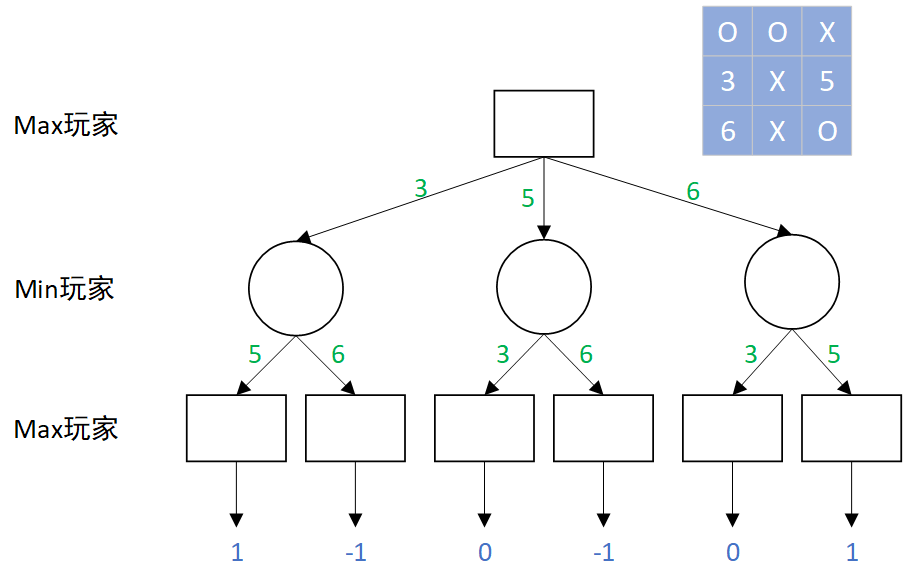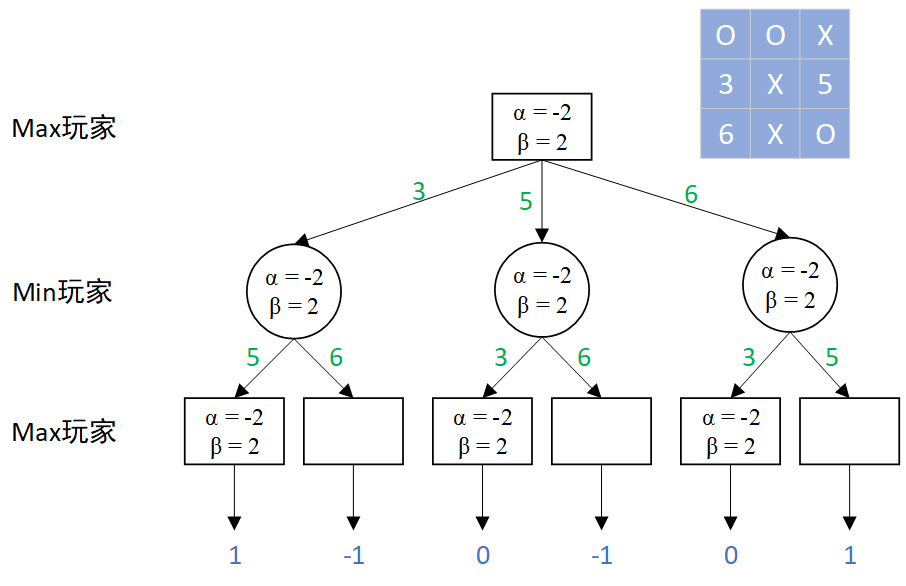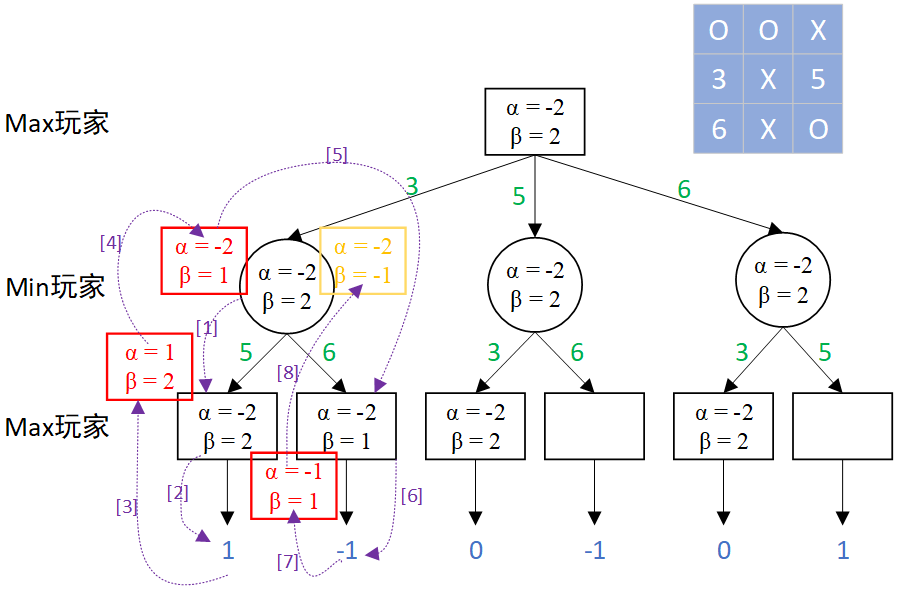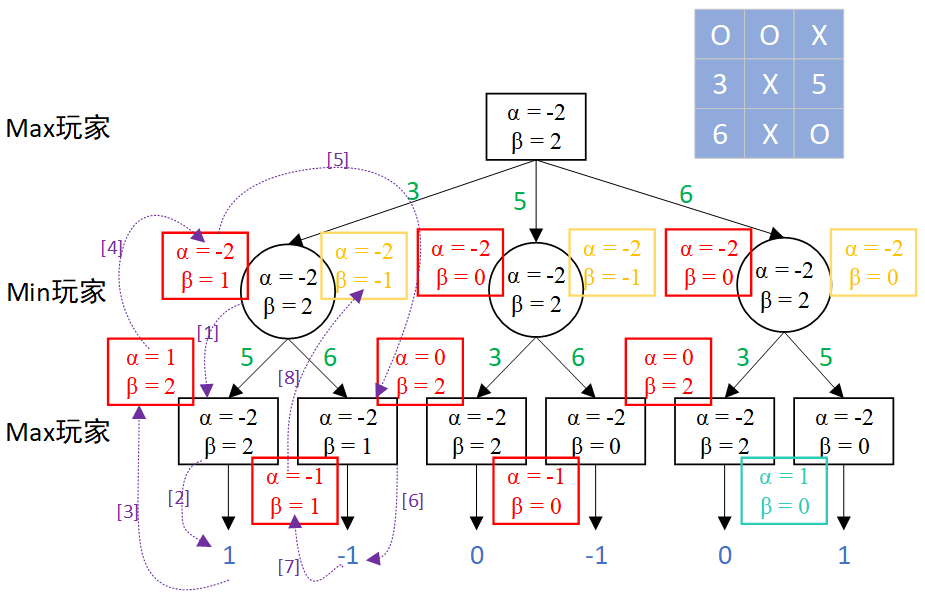## 一、对抗搜索简介

对抗搜索也称为博弈搜索，在一个竞争的环境中，智能体之间通过竞争实现相反的利益，一方最大化这个利益，另外一方最小化这个利益。
最小最大搜索(Minimax Search)是对抗搜索中最为基本的方法，给定一个游戏搜索树，Minimax算法通过每个节点的Minimax值来决定最优策略，当然，MAX希望最大化Minimax值，而MIN则相反。Minimax是一种简单有效的对抗搜索手段 ，但是如果搜索树极大，则无法在有效时间内返回结果。
Alpha-Beta剪枝搜索(Pruning Search)是一种对Minimax进行改进的算法，即在搜索过程中可剪除无需搜索的分支节点，且不影响搜索结果。

## 二、Alpha-Beta剪枝搜索图解

### 1.总体规则

α {\alpha} ：玩家MAX目前得到的最高收益；假设 n {n} 是MIN节点，如果 n {n} 的一个后续节点可提供的收益小于𝛼，则 n {n} 及其后续节点可被剪枝。
β {\beta} ：玩家MIN目前给对手的最小收益；假设 n {n} 是MAX节点，如果 n {n} 的一个后续节点可获得收益大于𝛽，则 n {n} 及其后续节点可被剪枝。
α {\alpha} β {\beta} 的值初始化分别设置为 − ∞ {-\infty} + ∞ {+\infty}
搜索过程即为：每个节点有两个值，分别是 α {\alpha} β {\beta} 。节点的 α {\alpha} β {\beta} 值在搜索过程中不断变化。其中， α {\alpha} 从负无穷大( − ∞ {-\infty} )逐渐增加、 β {\beta} 从正无穷大( + ∞ {+\infty} )逐渐减少。如果一个节点中 α {\alpha} > β {\beta} ，则该节点的后续节点可剪枝。

### 2.结合井字棋图解Alpha-Beta剪枝搜索

上面的规则可能有点抽象，下面我将从人机井字棋对战来图解Alpha-Beta剪枝搜索；
首先我们需要进行一些设置：
(1)电脑：Max玩家，其落子符号表示为“O”，落子数值表示为“1”；
(2)人类：Min玩家，其落子符号表示为“X”，落子数值表示为“-1”；
(3)棋盘最终为电脑赢，则得分为1，若为人类赢，则得分为-1，若为平局，得分为0；
(4)由于棋盘最终得分只有三种情况-1、0、1，那么 α {\alpha} 初始值可设为-2， β {\beta} 初始值可设为2；

假设我们的棋局到了图中蓝色九宫格的情况，现在还剩三个空位置：3、5、6号位可以落子，下一步该Max玩家(电脑)落子，此时，电脑就要思考了，我下哪一个位置更有利呢？
如果电脑落子为3，交换玩家后，人类落子为5，那么电脑最后落子为6，那么电脑将会胜利，如此电脑将会计算之后所有的落子情况。如下图所示，我们可以看到，当电脑这一步落子为6时，最后的结果是电脑胜利或者平局，相比于其他两种存在人类胜利的情况来说，落子为6当是最佳选择，但是怎么样让电脑知道6是最佳选择呢？
图中方框代表Max节点，圆圈代表Min节点，绿色数字代表此时选择落子的位置，蓝色数字代表最终棋盘得分，-1代表Min玩家胜利，1代表Max玩家胜利，0代表平局。此时我们的 α {\alpha} β {\beta} 就派上用场了！
每个节点设置有 α {\alpha} β {\beta} 值，在搜索中不断更新。
更新规则为：
(1)Max节点，只更新 α {\alpha} 值，更新为Max(当前 α {\alpha} ，下一节点 α {\alpha} ，下一节点 β {\beta} )；
(2)Min节点，只更新 β {\beta} 值，更新为Min(当前 β {\beta} ，下一节点 α {\alpha} ，下一节点 β {\beta} )；
(3)更新顺序为先左支向下传递，后左支向上更新，再右支向下传递，后右支向上更新；、步表示左支向下传递，直接将 α {\alpha} β {\beta} 值向下传递到最后一个节点；
、步表示左支向上更新：
Max节点只更新 α {\alpha} ，Max(当前 α {\alpha} =-2，下一层只有1) = 1 ——> α {\alpha} =1 ， β {\beta} =2
Min节点只更新 β {\beta} ，Min(当前 β {\beta} =2，下一层 α {\alpha} =1，下一层 β {\beta} =2) = 1 ——> α {\alpha} =-2 ， β {\beta} =1
、步表示右支向下传递，直接父节点 α {\alpha} β {\beta} 更新后的值向下传递到最后一个节点；
、步表示右支向上更新：
Max节点只更新 α {\alpha} ，Max(当前 α {\alpha} =-2，下一层只有-1) = -1 ——> α {\alpha} =-1 ， β {\beta} =1
Min节点只更新 β {\beta} ，Min(当前 β {\beta} =1，下一层 α {\alpha} =-1，下一层 β {\beta} =1) = -1 ——> α {\alpha} =-2 ， β {\beta} =-1同理，我们可以按照上面的流程得到所有节点的更新值，最后得到Min层，三个节点的黄色更新框。
对于最顶层的Max节点来说，它需要选择Min层中 β {\beta} 最大的那个节点对应的选项，这样可以给对手造成最小的收益。
(1)选择3号位： β {\beta} = -1
(2)选择5号位： β {\beta} = -1
(3)选择6号位： β {\beta} = 0
由于对手是Min玩家，需要 β {\beta} 值越小越好，那么Max玩家要使Min玩家收益最小，就应当选择 β {\beta} 最大的节点，即电脑此时应当选择6号位。
图中的青色方框显示的是 α {\alpha} β {\beta} 的情况，如果该节点下面还有节点，就可以进行剪枝，即，其下面的节点不必再进行搜索了。## 三、井字棋python实现

按照上述的搜索流程，我们就可以进行人机井字棋对战了。实际搜索树会更复杂一些，上面的图解是简化到只剩3步的情况，方便大家理解 α {\alpha} β {\beta} 更新的过程。

import random
import numpy as np

# 棋盘显示函数,每次落子后显示棋盘
def show(chessboard):
for i in range(len(chessboard)):
mark = ' '
row = chessboard[i]
for j in row:
mark = mark + tag[j + 1] + ' '
print(mark)

# 判断是否产生赢家
def terminal(chessboard, win, position):
for line in win:
m1,n1 = position[line],position[line]
m2,n2 = position[line],position[line]
m3,n3 = position[line],position[line]
if chessboard[m1][n1] == chessboard[m2][n2] == chessboard[m3][n3] == -1:
return -1
elif chessboard[m1][n1] == chessboard[m2][n2] == chessboard[m3][n3] == 1:
return 1
return 0

# 判断棋盘是否还有空位
def empty(chessboard, position):
for point in position:
if chessboard[point][point] == 0:
return True
return False

# 电脑走位之后,交换玩家,直到棋盘出现赢家或者没有空位
def alpha_beta(chessboard, win, position, now_player, next_player, alpha, beta):

winner = terminal(chessboard, win, position)
if winner != 0:
return winner
elif empty(chessboard, position) == False:
return 0

for i in range(len(position)):
temp = position[i]
if chessboard[temp][temp] == 0:
chessboard[temp][temp] = now_player

test = alpha_beta(chessboard, win, position, next_player, now_player, alpha, beta)

chessboard[temp][temp] = 0

# 如果现在是电脑,Max玩家,修改alpha值
if now_player == 1:
if test > alpha:
alpha = test
if alpha >= beta:
return alpha

# 如果现在是玩家,Min玩家,修改beta值
else:
if test < beta:
beta = test
if alpha >= beta:
return alpha

# 修改上一个节点的值
if now_player == 1:
node = alpha
else:
node = beta
return node

# 电脑计算目前最优的位置
def computer_move(chessboard, win, position):

val = -2
move_ = []
for i in range(len(position)):
temp = position[i]
# 检查所有可走的位置
if chessboard[temp][temp] == 0:
chessboard[temp][temp] = 1   # 假设电脑走该位置

if terminal(chessboard, win, position) == 1:
return temp, i

test = alpha_beta(chessboard, win, position, -1, 1, -2, 2)
chessboard[temp][temp] = 0   # 将该位置清0

if test > val:
val = test
move_ = [temp]
elif test == val:
move_.append(temp)

cmove = random.choice(move_)
for j in range(len(position)):
if cmove == position[j]:
return cmove, j

if __name__ == '__main__':
chessboard = [[0,0,0],[0,0,0],[0,0,0]]
position = [[0,0],[0,1],[0,2],[1,0],[1,1],[1,2],[2,0],[2,1],[2,2]]
win = ((0, 1, 2), (3, 4, 5), (6, 7, 8),
(0, 3, 6), (1, 4, 7), (2, 5, 8),
(0, 4, 8), (2, 4, 6))
tag = ['x', '.', 'o']
result = ['玩家胜利!', '平局!', '电脑胜利!']

player = -1     # 玩家为Min玩家
computer = 1    # 电脑为Max玩家

first = input("请选择哪一方先下,输入x表示玩家先下,输入o表示电脑先下:")
if first == "x":
next_move = player
elif first == "o":
next_move = computer
else:
next_move = player
print("输入有误,默认玩家先下")

show(chessboard)

print('=========================')
print('游戏开始!')
while empty(chessboard, position) and terminal(chessboard, win, position) == 0:

if next_move == player and empty(chessboard, position):
try:
hmove = int(input("请选择你的落子位置(0-8):"))
if chessboard[position[hmove]][position[hmove]] != 0:
print('该位置已有棋子,请重新选择')
continue
chessboard[position[hmove]][position[hmove]] = player
next_move = computer
except:
print("输入为0~8,请重试")
continue

if next_move == computer and empty(chessboard, position):
cmove, po = computer_move(chessboard, win, position)
chessboard[cmove][cmove] = computer
print("电脑落子为:", po)
next_move = player

show(chessboard)

print('=========================')
print('最终棋局:')
show(chessboard)
s = terminal(chessboard, win, position)
print('游戏结束:',result[s+1])
print('=========================')


请选择哪一方先下,输入x表示玩家先下,输入o表示电脑先下:x
. . .
. . .
. . .
=========================

. . .
. o .
. . x

. . o
. o x
. . x

. . o
. o x
x o x

. x o
o o x
x o x

x x o
o o x
x o x
=========================

x x o
o o x
x o x

=========================


请选择哪一方先下,输入x表示玩家先下,输入o表示电脑先下:o
. . .
. . .
. . .
=========================

. . .
. . .
o . .

o x .
. . .
o . .

o x .
o x .
o . .
=========================

o x .
o x .
o . .

=========================